# The Companion IC

The Companion IC is a very cool looking Clock + Ohmmeter + Capacitance Meter + Diode Tester!

BeginnerFull instructions provided6,169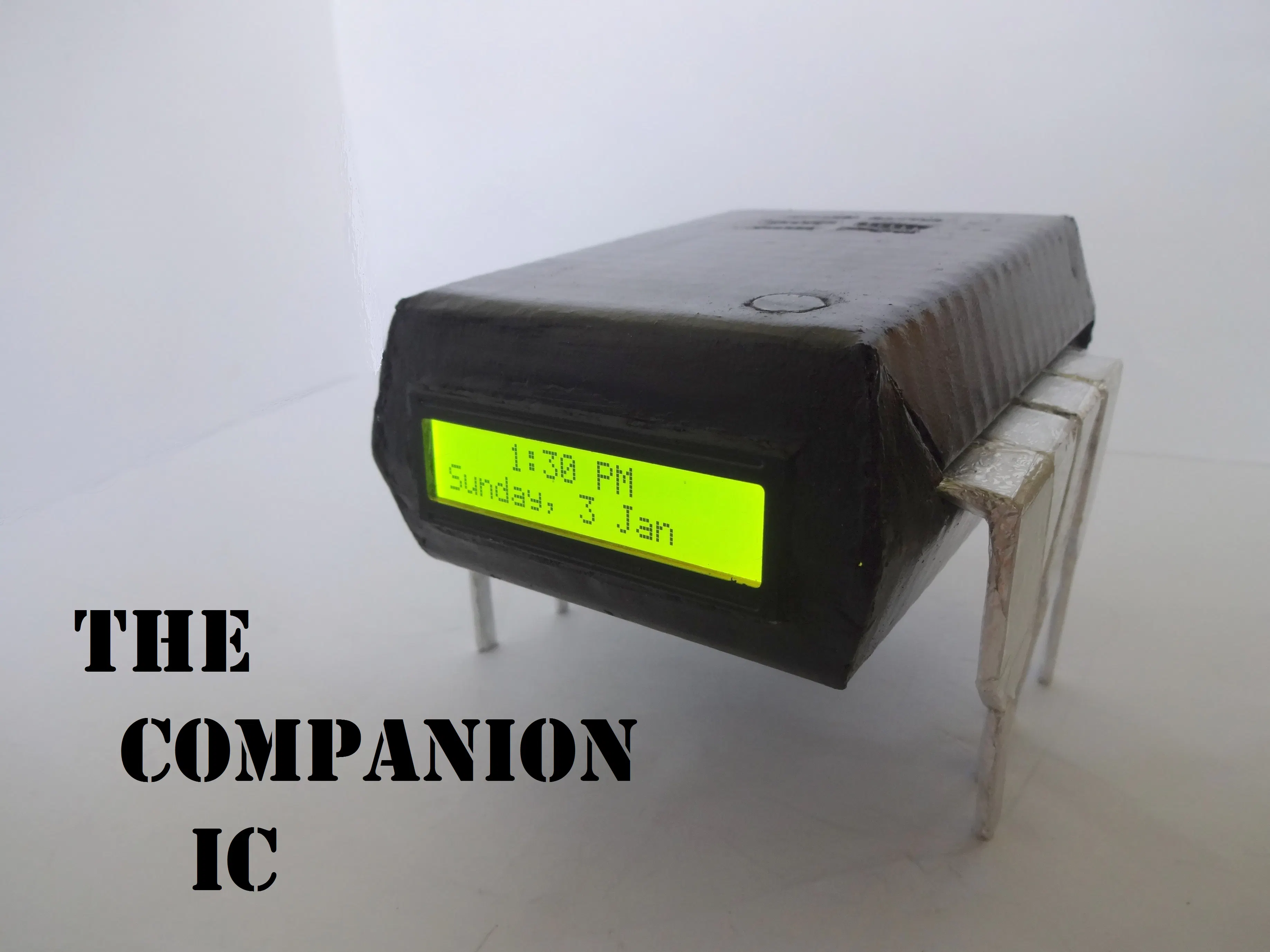## Things used in this project

### Hardware componentsArduino Nano R3
×1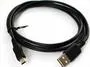USB-A to Mini-USB Cable
×1Resistor 10k ohm 2x 220 ohm resistor 1x (1k, 4.7k, 47k, 100k) ohm resistors
×4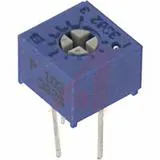Single Turn Potentiometer- 10k ohms
×1SparkFun Pushbutton switch 12mm
×2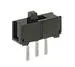Slide Switch
×3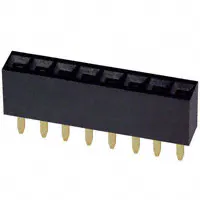Female Header 8 Position 1 Row (0.1")
×4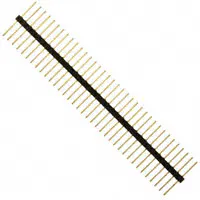Male-Header 36 Position 1 Row- Long (0.1")
×2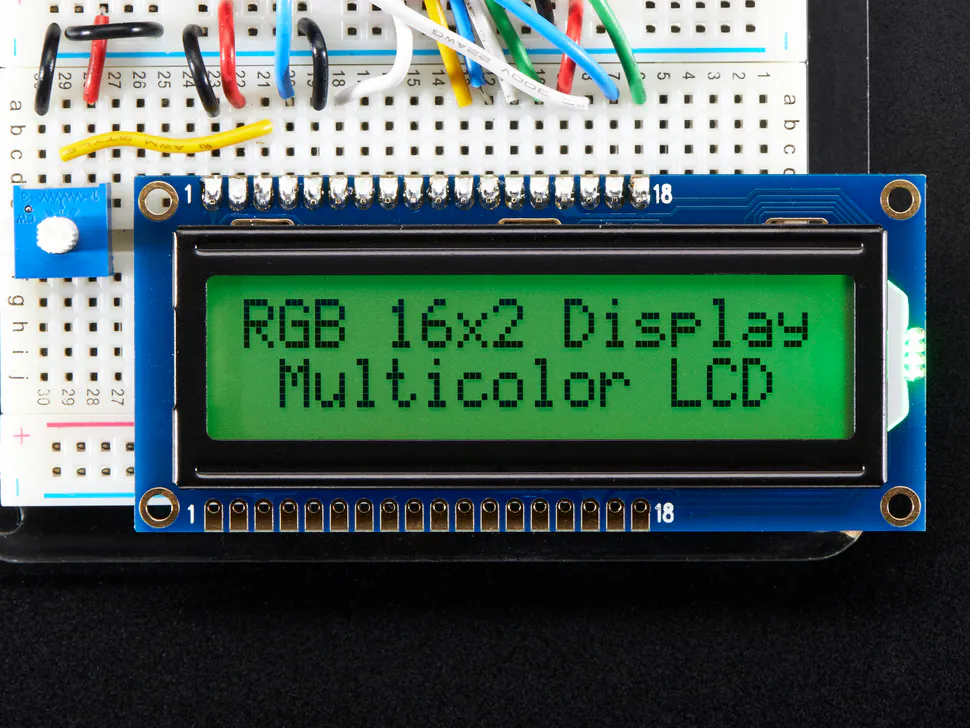Adafruit RGB Backlight LCD - 16x2
×1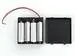4xAA battery holder
×1

### Hand tools and fabrication machinesSoldering iron (generic)Hot glue gun (generic)

## Code

### Code snippet #1

Plain text
```//Analog pin used to find resistance

int Apin=7;

//values of r1 to r5
float r1=1000;
float r2=4700;
float r3=10000;
float r4=47000;
float r5=100000;

//pins of r1 to r5
int r1_pin=2;
int r2_pin=3;
int r3_pin=4;
int r4_pin=5;
int r5_pin=6;

float R=0;       //calculate unknown and store here

String finalR;   //final value to be displayed along with units

int caseno;   //for debugging, stores the case number
// we divide the entire range into cases and assign each a number,
// total 5 cases
// case1 : less than 2850
// case2 : 2850  to   7350
// case3 : 7350  to   28500
// case4 : 28500 to   73500
// case5 : more than 73500

#include <stdlib.h> // needed for converting float to string,
//has the String(float,n) function. Explained below.

void setup() {
Serial.begin(9600);

}

void loop() {

//first we find unknown resistance using 1kOhm resistor
//Therefore, disable R2, R3, R4 and R5
digitalWrite(r2_pin, LOW);   //turn each pin to LOW before setting it as INPUT
pinMode(r2_pin, INPUT);      // turning it to INPUT when its HIGH enables the
// internal pullup resistor

digitalWrite(r3_pin, LOW);
pinMode(r3_pin, INPUT);

digitalWrite(r4_pin, LOW);
pinMode(r4_pin, INPUT);

digitalWrite(r5_pin, LOW);
pinMode(r5_pin, INPUT);

pinMode(r1_pin, OUTPUT);
digitalWrite(r1_pin, HIGH);

// if value < 2850, finalR = value(using 1kOhm)
if(R<2850){

caseno=1;

if(R<1000){ //if value less than 1000 use "Ohm" not "kOhm"
finalR = String(R,2) + "Ohm";  //String(float,n) Converting float to string
//with n digits after decimal
// attach "Ohm" after value to the string,
//'+' joins two strings here
}
else{ //use "kOhm
R=R/1000;
finalR = String(R,2) + "kOhm";
}
}

//if value between 2850 and 7350 , use value obtained by 4.7kOhm
else if(R>=2850 && R<7350){
caseno=2;

digitalWrite(r1_pin, LOW);  //Enable only 4.7kOhm
pinMode(r1_pin, INPUT);

digitalWrite(r3_pin, LOW);
pinMode(r3_pin, INPUT);

digitalWrite(r4_pin, LOW);
pinMode(r4_pin, INPUT);

digitalWrite(r5_pin, LOW);
pinMode(r5_pin, INPUT);

pinMode(r2_pin, OUTPUT);
digitalWrite(r2_pin, HIGH);

finalR = String(R,2) + "kOhm";

}

//if value between 7350 and 28500, use value obtained by 10kOhm
else if(R>=7350 && R<28500){
caseno=3;

digitalWrite(r1_pin, LOW);
pinMode(r1_pin, INPUT);

digitalWrite(r2_pin, LOW);
pinMode(r2_pin, INPUT);

digitalWrite(r4_pin, LOW);
pinMode(r4_pin, INPUT);

digitalWrite(r5_pin, LOW);
pinMode(r5_pin, INPUT);

pinMode(r3_pin, OUTPUT);
digitalWrite(r3_pin, HIGH);

finalR= String(R,2) + "kOhm";
}

//if value between 28500 and 73500, use value obtained by 47kOhm
else if(R>=28500 && R<73500){
caseno=4;

digitalWrite(r1_pin, LOW);
pinMode(r1_pin, INPUT);

digitalWrite(r2_pin, LOW);
pinMode(r2_pin, INPUT);

digitalWrite(r3_pin, LOW);
pinMode(r3_pin, INPUT);

digitalWrite(r5_pin, LOW);
pinMode(r5_pin, INPUT);

pinMode(r4_pin, OUTPUT);
digitalWrite(r4_pin, HIGH);

finalR = String(R,2) + "kOhm";

}

//if value more than 73500, use value obtained by 100kOhm
else if(R>=73500){
caseno=5;

digitalWrite(r1_pin, LOW);
pinMode(r1_pin, INPUT);

digitalWrite(r2_pin, LOW);
pinMode(r2_pin, INPUT);

digitalWrite(r3_pin, LOW);
pinMode(r3_pin, INPUT);

digitalWrite(r4_pin, LOW);
pinMode(r4_pin, INPUT);

pinMode(r5_pin, OUTPUT);
digitalWrite(r5_pin, HIGH);

finalR = String(R,2) + "kOhm" ;

}

Serial.println(finalR); //printing the final string with units
Serial.println(" ");

delay(1000);

}
```

### Code snippet #2

Plain text
```/*  RCTiming_capacitance_meter
*  code concept taken from Paul Badger 2008
*
*    The capacitor's voltage at one time constant is defined as
*    63.2% of the charging voltage.
*    i.e, A Capacitor is filled to 63.2% of its total capacity in
*    1 Time Constant
*/

int analogPin=0 ;         // analog pin for measuring capacitor voltage
int chargePin=7 ;        // pin to charge the capacitor - connected to
// one end of the charging resistor
int dischargePin=12 ;        // pin to discharge the capacitor,
// same used for diode test(checkPin1)
float resistorValue=10000.0;   // We use 10kOhm resistor

unsigned long startTime;
unsigned long elapsedTime;
float microFarads;                // floating point variable to preserve precision

void setup(){

pinMode(chargePin, OUTPUT);     // set chargePin to output
digitalWrite(chargePin, LOW);

Serial.begin(9600);             // initialize serial transmission for debugging
}

void loop(){

digitalWrite(chargePin, HIGH);  // set chargePin HIGH and capacitor charging
startTime = millis();

while(analogRead(analogPin) < 648){  // just wait and do nothing till 648
// 647 is 63.2% of 1023,
// which corresponds to full-scale voltage
}

elapsedTime= millis() - startTime;
// convert milliseconds to seconds ( 10^-3 )

microFarads = ((float)elapsedTime / resistorValue) * 1000;
// (float) converts "unsigned long" elapsed time to float

Serial.print(elapsedTime);       // print the value to serial port
Serial.print(" mS    ");         // print units

Serial.print((long)microFarads);       // print the value to serial port
}
else
{

Serial.print((long)nanoFarads);    // print the value to serial port
}

/* dicharge the capacitor  */
digitalWrite(chargePin, LOW);           // set charge pin to  LOW
pinMode(dischargePin, OUTPUT);          // set discharge pin to output
digitalWrite(dischargePin, LOW);        // set discharge pin LOW
while(analogRead(analogPin) > 0){       // wait until capacitor is completely discharged
}

pinMode(dischargePin, INPUT);           // set discharge pin back to input
}
```

### Code snippet #3

Plain text
```String state = "null"; //prints "null" for reverse bias or nothing connected

int checkPin1 = 12;
int checkPin2 = 6;

void setup() {
Serial.begin(9600);

}

void loop() {

pinMode(checkPin1, OUTPUT);
digitalWrite(checkPin1, LOW); //pin 11 is set to low

//analog read is normally pulled up by the 10k resistor, so null reading is 1023
//In forward bias, the analog pin gets connected to checkPin1, which is LOW. So reading less than 1023
//Practically a small current flows in reverse bias as well, so we take 700 to differentiate

state="forward";

}

Serial.println(state);
state = "null";
delay(500);

}
```

### Code snippet #4

Plain text
```// Date and time functions using a DS1307 RTC connected via I2C and Wire lib
#include
#include

RTC_DS1307 rtc;//creating "rtc" object of RTC_DS1307, objects are used to access functions
//more on objects and classes: https://www.youtube.com/watch?v=ABRP_5RYhqU

char daysOfTheWeek = {"Sunday", "Monday", "Tuesday", "Wednesday", "Thursday", "Friday", "Saturday"};

void setup () {

Serial.begin(9600);
rtc.begin();

// following line sets the RTC to the date & time this sketch was compiled

// This line sets the RTC with an explicit date & time, for example to set
// January 21, 2014 at 3am you would call:
// rtc.adjust(DateTime(2014, 1, 21, 3, 0, 0));
}

void loop () {
DateTime now = rtc.now();

Serial.print(now.year());
Serial.print('/');
Serial.print(now.month());
Serial.print('/');
Serial.print(now.day());
Serial.print(" (");
Serial.print(daysOfTheWeek[now.dayOfTheWeek()]);
Serial.print(") ");
Serial.print(now.hour());
Serial.print(':');
Serial.print(now.minute());
Serial.print(':');
Serial.print(now.second());
Serial.println();

Serial.println();
delay(1000);
}
```